## Relative velocity

9-17-99

Section 3.8

### Relative velocity in 1 dimension

Most people find relative velocity to be a relatively difficult concept. In one dimension, however, it's reasonably straight-forward. Let's say you're walking along a road, heading west at 8 km/hr. A train track runs parallel to the road and a train is passing by, traveling at 40 km/hr west. There is also a car driving by on the road, going 30 km/hr east. How fast is the train traveling relative to you? How fast is the car traveling relative to you? And how fast is the train traveling relative to the car?

One way to look at it is this: in an hour, the train will be 40 km west of where you are now, but you will be 8 km west, so the train will be 32 km further west than you in an hour. Relative to you, then, the train has a velocity of 32 km/hr west. Similarly, relative to the train, you have a velocity of 32 km/hr east.

Using a subscript y for you, t for the train, and g for the ground, we can say this: the velocity of you relative to the ground =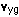= 8 km/hr west the velocity of the train relative to the ground == 40 km/hr west

Note that if you flip the order of the subscripts, to get the velocity of the ground relative to you, for example, you get an equal and opposite vector. You can write this equal and opposite vector by flipping the sign, or by reversing the direction, like this: the velocity of the ground relative to you =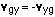= -8 km/hr west = 8 km/hr east

The velocity of the train relative to you,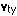, can be found by adding vectors appropriately. Note the order of the subscripts in this equation: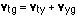Rearranging this gives: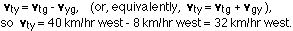A similar argument can be used to show that the velocity of the car relative to you is 38 km/hr east. the velocity of you relative to the ground == 8 km/hr west = -8 km/hr east the velocity of the car relative to the ground =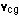= 30 km/hr east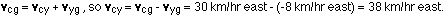The velocity of the train relative to the car is 70 km/hr west, and the velocity of the car relative to the train is 70 km/hr east.

### Relative velocity in 2 dimensions

In two dimensions, the relative velocity equations look identical to the way they look in one dimension. The main difference is that it's harder to add and subtract the vectors, because you have to use components. Let's change the 1D example to 2D. The train still moves at 40 km/hr west, but the car turns on to a road going 40° south of east, and travels at 30 km/hr. What is the velocity of the car relative to the train now?

The relative velocity equation for this situation looks like this: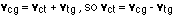The corresponding vector diagram looks like this: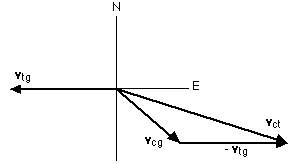Because this is a 2-D situation, we have to write this as two separate equations, one for the x-components (east-west) and one for the y-components (north-south):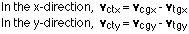Now we have to figure out what the x and y components are for these vectors. The train doesn't have a y-component, because it is traveling west. So: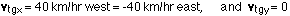The car has both an x and y component: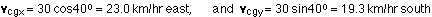Plugging these in to the x and y equations gives: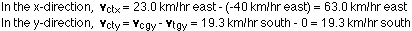Combining these two components into the vector gives a magnitude of: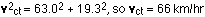at an angle given by the inverse tangent of 19.3 / 63.0, which is 17 degrees. So, the velocity of the car relative to the train is 66 km/hr, 17 degrees south of east.